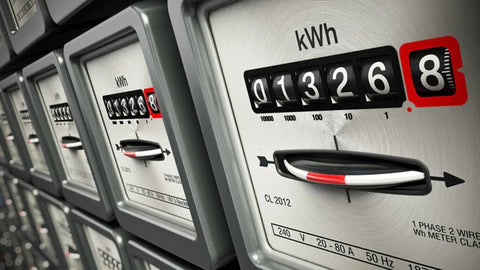Electricity Cost Calculator | kWh Cost Calculator - ShopSolar.com

# Electricity Cost Calculator | kWh Cost Calculator

Ever wonder what exactly your local power company is charging you for at the end of every month and how those values are calculated?

Let’s look at how an electricity cost calculator (kWh cost calculator) can help us in the world of solar power, but first, what are kilowatt-hours?

## Electricity Cost Calculator (kWh Cost Calculator)

Power consumption
W
milliwatts (mW)
watts (W)
kilowatts (kW)
megawatts (MW)
gigawatts (GW)
British thermal units per hour (BTU/h)
mechanical horsepowers (hp(l))
Energy price
\$ / kWh
Usage time
hours /
day
day (day)
week (wk)
month (mo)
year (yr)
Power consumed
kWh /
day
day (day)
week (wk)
month (mo)
year (yr)
Cost
\$ /
yr
day (day)
week (wk)
month (mo)
year (yr)

## What Is kWh?The easiest way to understand the concept of kilowatt-hours is to use an everyday example. So, let’s say we have a 70 W lightbulb in our garage.

Over 60 minutes, that lightbulb uses 70 W of energy. To make life simpler, we say the lightbulb consumes 70 watt-hours of energy.

1,000 watt-hours gives us 1 kilowatt-hour, and this is how kWh to kW calculators work.

On average, an American household uses 500 to 1,000 kWh every month. When you decide to install a solar system, the energy it produces is also measured in kW and kWh.

The most common place you find this measurement is on your power bill. Every kWh of power is the ‘unit’ you’re being charged for.

One of the main driving forces behind installing solar is to have a net zero usage at the end of the month.

This means that your energy production is equivalent to your consumption, leaving you with little to no credit that needs to be paid off.

But how do the local energy companies charge the consumers?

## Calculating the Cost Electricity (kWh Cost)

Whether it’s a kW to amps calculator, a kWh cost calculator, or an electricity cost calculator, it’s best to start with smaller and singular examples before factoring in the entire month’s worth of appliances.### Cost Per Appliance

So, let’s say we have a microwave that has a power rating of 1,500 W and we’ve used it for around 10 minutes.

When you pay for electricity, you pay per kilowatt hour. In most cases, your appliances are rated in watts. Changing that value to kilowatts is simply a matter of multiplying by 1,000.

The cost per kilowatt-hour depends on the state you live in. For this example, we’ll use the national average of 0.23 cents per kilowatt-hour.

This might not seem like a large amount of money, but we’re only going to be calculating a single appliance.

This calculator typically follows a simple 3-step protocol; however, some steps won’t be necessary.

### Step 1 – The Conversion

We want to work with kilowatts instead of watts, and most appliances are rated using the latter.

So, if we take our 1,500 W microwave, we’re left with 1.5 kW.

Once you’ve understood the kW to watts conversions, you always have the correct values to work with.

### Step 2 – The Calculation

Now we need to determine the number of kilowatt-hours this appliance uses with the values we’re given.

If we want to find kilowatt-hours, we need to change our minutes into hours. Converting the 10 minutes into hours gives us 0.167 hours.

So now we have the kilowatts and the hours, multiply them together and voila: we get kilowatt hours.

This leaves us with around 0.25 kWh.

These values are flexible, and you can easily convert kWh to kW if need be.

### Step 3 – The Cost

The final step is to figure out how money this microwave will cost us if it’s using 0.25kWh.

Simply take the kilowatt hours and multiply it by the 0.23 cents from earlier. This leaves us with a value of around 0.06 cents.

Keep in mind that this is for a singular appliance and only for the ten minutes it was operating. Every appliance in your household uses a different amount of power.

These calculators take the individual appliances, calculate all the appliances’ kWh, and sum them up to produce a net cost.

If you want to work this out on a smaller scale, you need to convert kWh to watts.

## Conclusion

Knowing how to use an electricity cost calculator (kWh calculator) helps you choose the size of your solar system. Once you know what your monthly consumption is, you can figure out the kWh per square foot you need to produce with the space you have available.You’ll be able to decide on an array of 150-watt or 200-watt solar panels once you know the output cost of your appliances.

You can also make use of our solar load calculator to determine your needs.

You can find all of the solar & electrical calculators we have created in order to help you plan, size and install your own solar power system here.

## Join the Solar Lab!